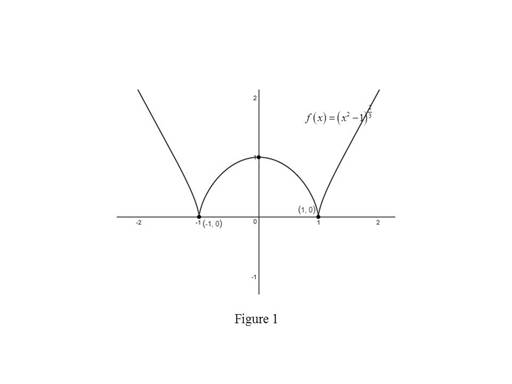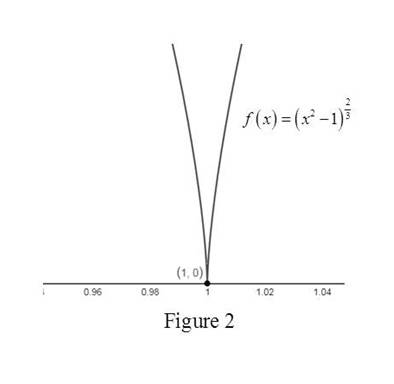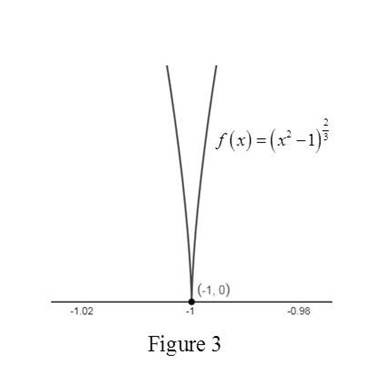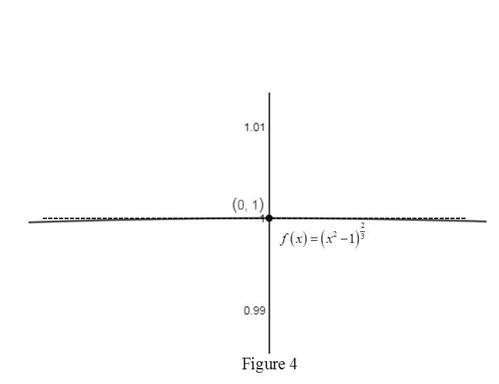# To graph: The function f ( x ) = ( x 2 − 1 ) 2 3 , and conclude the differentiability of the points ( 1 , 0 ) , ( 0 , 1 ) and ( − 1 , 0 ) .### Single Variable Calculus: Concepts...

4th Edition
James Stewart
Publisher: Cengage Learning
ISBN: 9781337687805### Single Variable Calculus: Concepts...

4th Edition
James Stewart
Publisher: Cengage Learning
ISBN: 9781337687805

#### Solutions

Chapter 2.7, Problem 40E
To determine

## To graph: The function f(x)=(x2−1)23, and conclude the differentiability of the points (1,0),(0,1) and (−1,0).

Expert Solution

The function f(x) is not differentiable at (1,0) and (1,0) but differentiable at (0,1).

### Explanation of Solution

Given:

The given function is f(x)=(x21)23.

Graph:

Use the online graphing calculator to draw the graph of the function f(x)=(x21)23 as shown below in Figure 1.Use the online graphing calculator to zoom toward the point (1,0) as shown below in Figure 2.From Figure 2, it is observed that when zoomed in the graph toward the point (1,0), the curve has high sharpness at (1,0). So, there is no unique tangent line at (1,0).

Thus, (1,0) is a corner point.

Hence, the given function f(x)=(x21)23 is not differentiable at (1,0).

Use the online graphing calculator to zoom toward the point (1,0) as shown below in Figure 3.From Figure 3, it is observed that when zoomed in the graph toward the point (1,0), the curve has high sharpness at (1,0). So, there is no unique tangent line at (1,0).

Thus, (1,0) is a corner point.

Hence, the function f(x)=(x21)23 is not differentiable at (1,0).

Use the online graphing calculator to zoom toward the point (0,1) as shown below in Figure 4.From Figure 4, it is observed that when zoomed in the graph toward the point (0,1), the curve and the tangent are indistinguishable.

It has a unique tangent.

Hence, the function f(x)=(x21)23 is differentiable at (0,1).

Conclusion:

By zooming the graph toward the point (1,0) and (1,0), the graph has no unique tangent line while zooming the graph toward the point (0,1), the graph has unique tangent line.

Thus, the function f(x)=(x21)23 is not differentiable at (1,0) and (1,0) but differentiable at (0,1).

### Have a homework question?

Subscribe to bartleby learn! Ask subject matter experts 30 homework questions each month. Plus, you’ll have access to millions of step-by-step textbook answers!